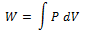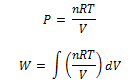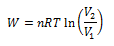A thermodynamic process is said to be taking place if the thermodynamic variable of the system change with time.

## Types of thermodynamic Process:-

1. Isothermal process – process taking place at constant temperature.
2. Adiabatic process – process where there is no exchange of heat.
3. Isochoric process – process taking place at constant volume
4. Isobaric process –Process taking place at constant Pressure.
5. Cyclic process – Process where the system returns to its original state.

Isothermal Process:

1. In isothermal process temperature of the system remains constant throughout the process.
2. For an isothermal process equation connecting P, V and T gives.

PV = constant
i.e., the pressure of given mass of gas varies inversely with its volume this is nothing but the Boyle’s law.

• In the isothermal process, there is no change in temperature, since internal energy for an ideal gas depends only on temperature hence in the isothermal process there is no change in internal energy. Thus,

ΔU=0

therefore,

ΔQ =ΔW

• Thus during isothermal process
Heat added (or subtracted) from the system = work done by (or on) the system

## Work done in Isothermal Process:

1. In an isothermal process, the temperature remains constant.
2. Consider pressure and volume of ideal gas changes from (P1, V1) to (P2, V2) then, from first law of thermodynamics

ΔW = PΔV

Now taking ΔV approaching zero i.e. ΔV and summing ΔW over entire process we get total work done by gas so we havewhere limits of integration goes from V1 to V2 as PV = n R T we havewhere limits of integration goes from V1 to V2 on integrating we get,Where n is the number of moles in a sample of gas taken.